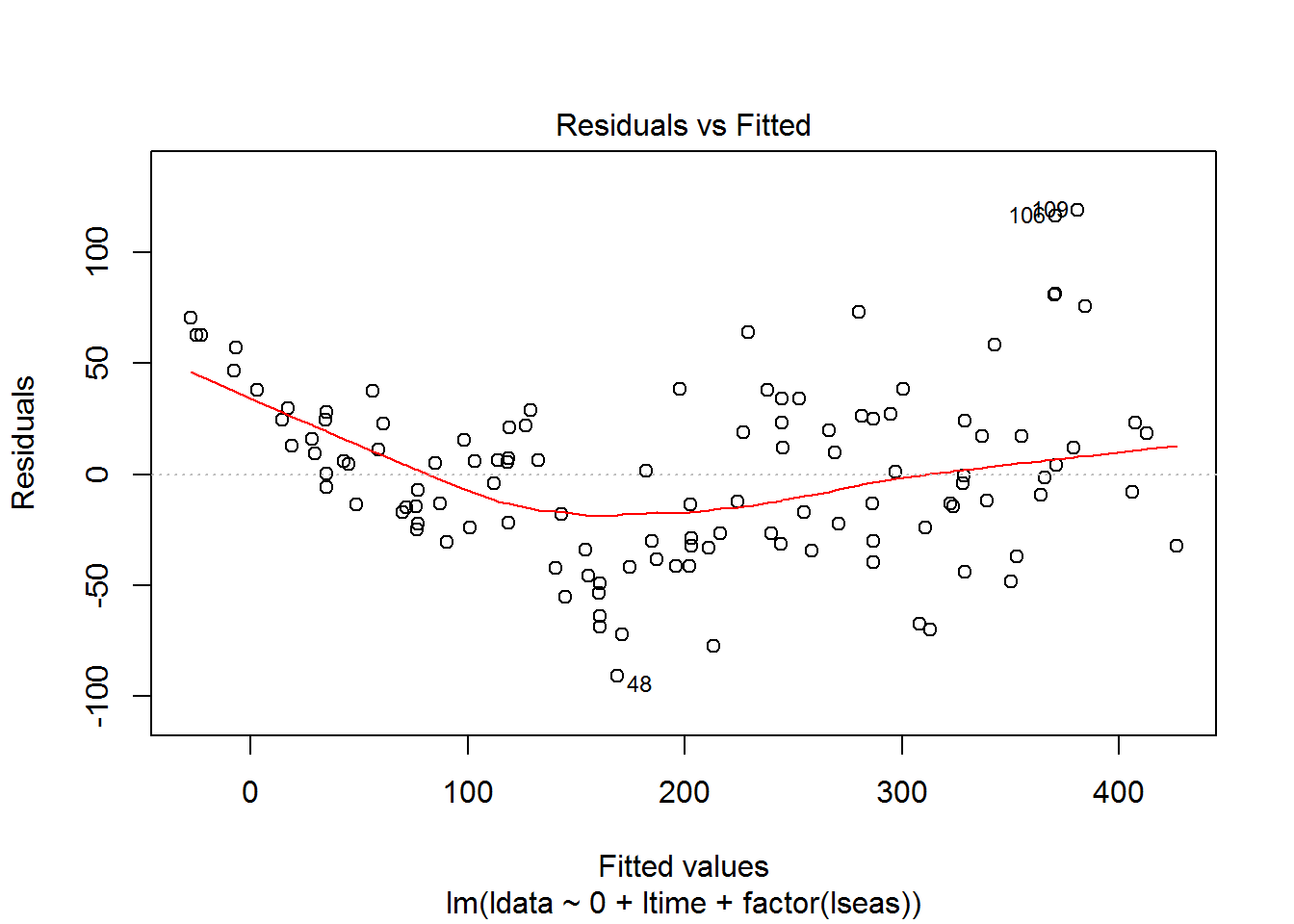``````###################################################
#
# Assignment 3
#
######################################################
#
#Under the file tab on R console, change the directory to your MA611 work directory
#Use the command dir() to see if Boston.dat is in the list of files
#Read the data and create a time series using the following commands ####
#### Analyzing time series data of robberies in Boston from Jan 1966 to Oct 1975 and forecasting for the months of Nov & Dec 1975. ####
## We would be using moving average decomposition, loess decomposition, Regression & Holt Winter Techniques
bosdata=scan(file='Boston.dat')
library(FinTS)``````
``## Warning: package 'FinTS' was built under R version 3.2.3``
``## Loading required package: zoo``
``## Warning: package 'zoo' was built under R version 3.2.3``
``````##
## Attaching package: 'zoo'``````
``````## The following objects are masked from 'package:base':
##
##     as.Date, as.Date.numeric``````
``````#To change the Boston.dat data into a ts object
tsbos=ts(bosdata,start=c(1966,1),frequency=12)
tsbos``````
``````##      Jan Feb Mar Apr May Jun Jul Aug Sep Oct Nov Dec
## 1966  41  39  50  40  43  38  44  35  39  35  29  49
## 1967  50  59  63  32  39  47  53  60  57  52  70  90
## 1968  74  62  55  84  94  70 108 139 120  97 126 149
## 1969 158 124 140 109 114  77 120 133 110  92  97  78
## 1970  99 107 112  90  98 125 155 190 236 189 174 178
## 1971 136 161 171 149 184 155 276 224 213 279 268 287
## 1972 238 213 257 293 212 246 353 339 308 247 257 322
## 1973 298 273 312 249 286 279 309 401 309 328 353 354
## 1974 327 324 285 243 241 287 355 460 364 487 452 391
## 1975 500 451 375 372 302 316 398 394 431 431``````
``````#
plot(tsbos)
## The above figure indicates that the robberies have been on the rise from 1966 to 1975
## After visual analysis, we could conclude the presence of Positive Trend and Seasonality.
## Further analysis to confirming these statements & for forecasts

str(bosdc)``````
``````## List of 6
##  \$ x       : Time-Series [1:118] from 1966 to 1976: 41 39 50 40 43 38 44 35 39 35 ...
##  \$ seasonal: Time-Series [1:118] from 1966 to 1976: 10.24 -4.84 -8.76 -28.96 -30.82 ...
##  \$ trend   : Time-Series [1:118] from 1966 to 1976: NA NA NA NA NA ...
##  \$ random  : Time-Series [1:118] from 1966 to 1976: NA NA NA NA NA ...
##  \$ figure  : num [1:12] 10.24 -4.84 -8.76 -28.96 -30.82 ...
##  \$ type    : chr "additive"
##  - attr(*, "class")= chr "decomposed.ts"``````
``````layout(1:4)
plot(tsbos)
plot(bosdc\$seasonal)
plot(bosdc\$trend)
plot(bosdc\$random)``````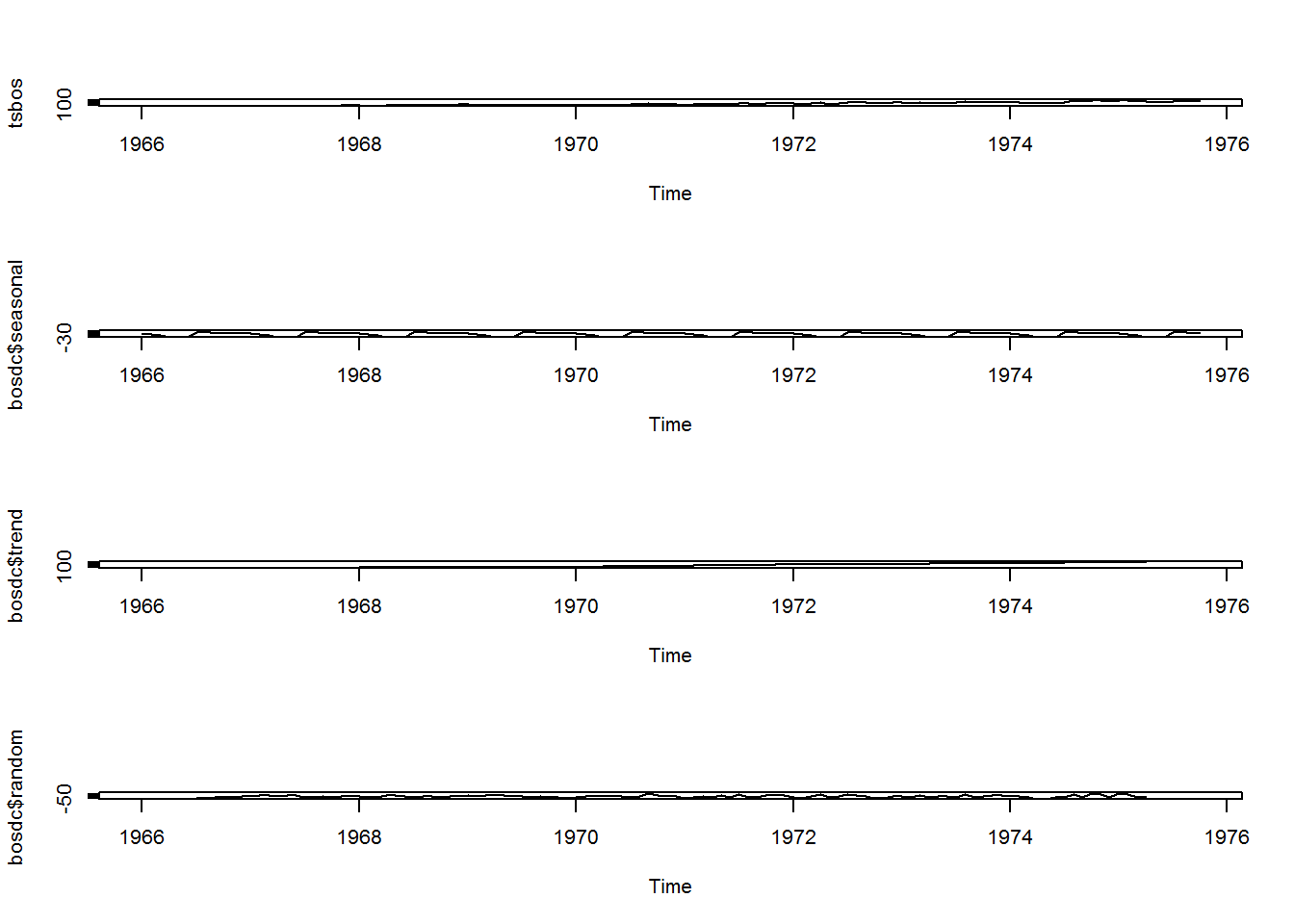``````## Above plots shows the robbery data series along with seasonal, trend & random components of the Additive Decomposition Model

layout(1:1)
plot(tsbos, lwd = 2)
lines(predad, col ="red", lwd = 2)``````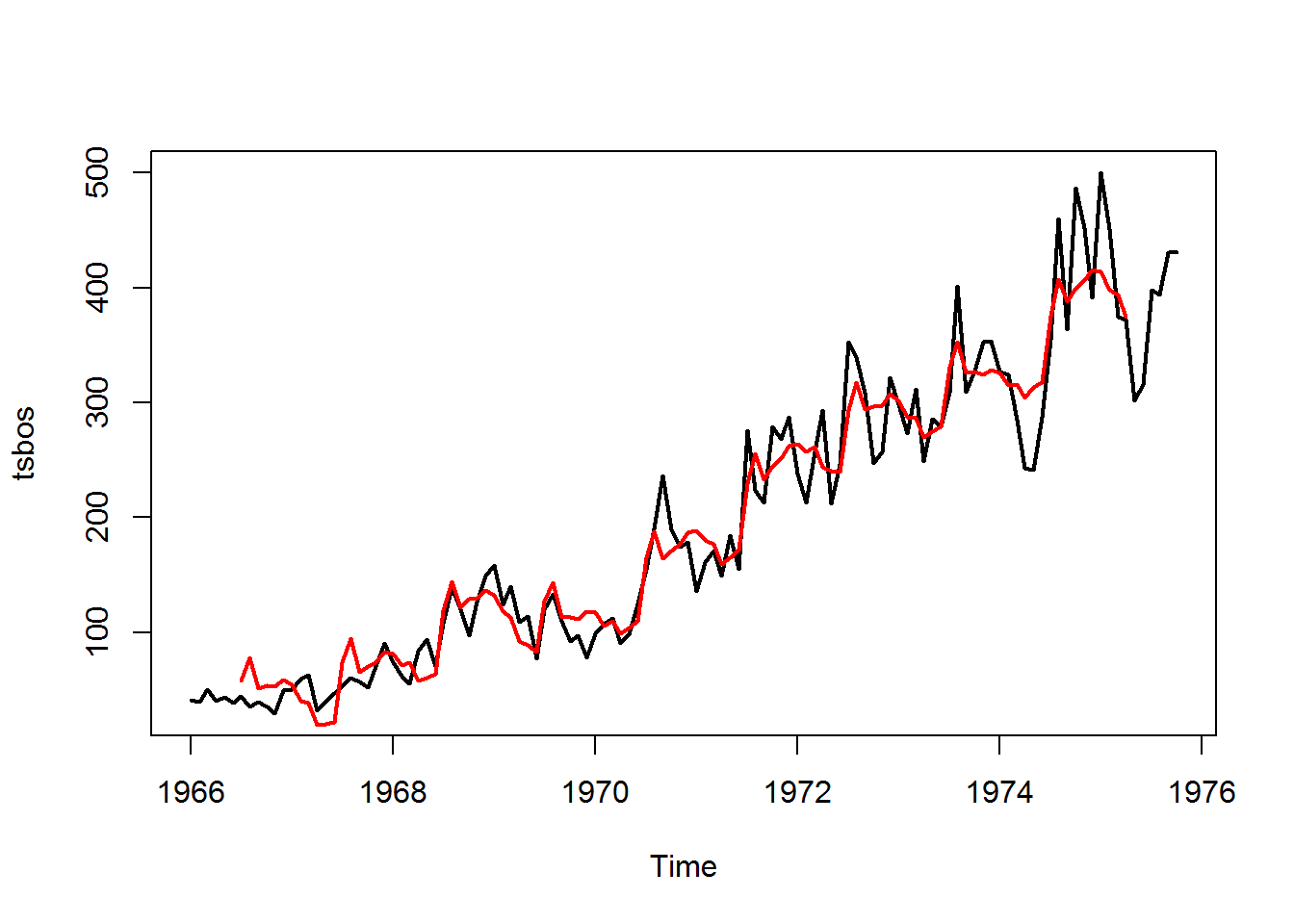``````#### fit seems to be bad for the seasonal peaks and as it worse as the trend increases, We can clearly see the overestimation at the lower tail & underestimation at the upper tail ####

bosadresid = window(bosdc\$random,start = c(1966, 7), end = c(1975, 4))
#### Removing some of the NA values from the data

``##  28.75867``
``````#### RMSE is found to be 28.75 for the Additive Model ####
abline(0,0)``````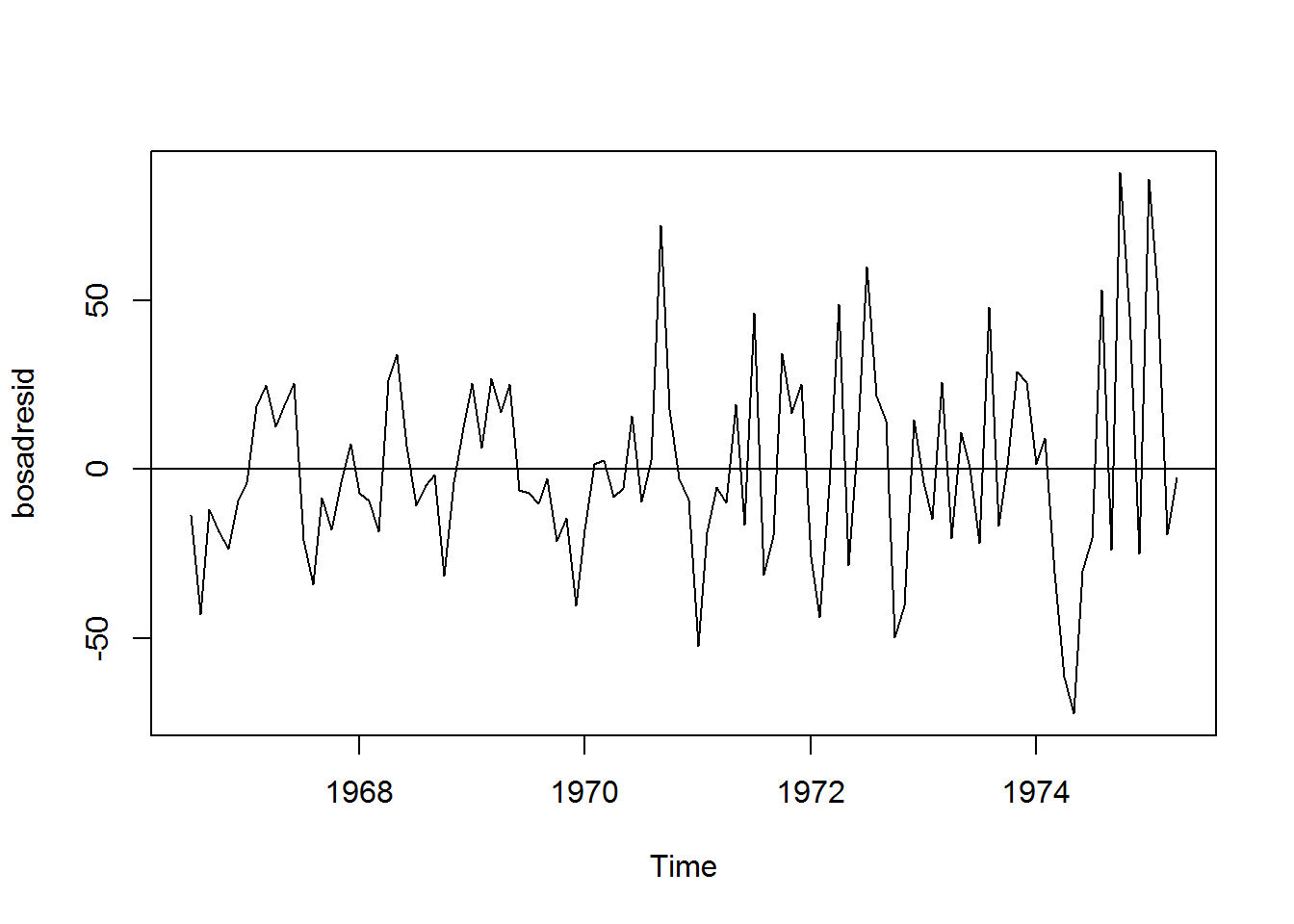``plot(bosdc\$random)``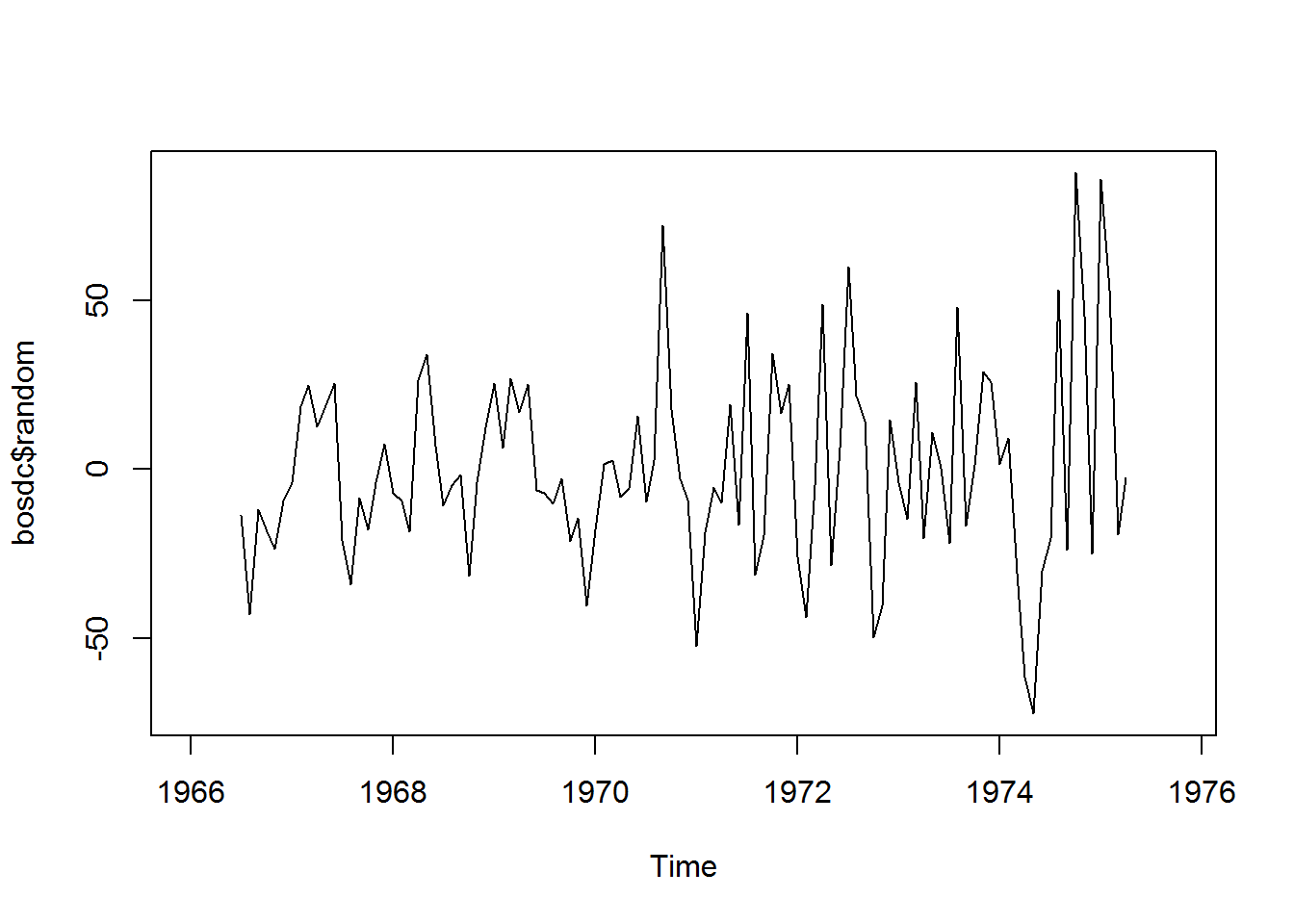``````## From the above plot, random fluctuations seems to increase with the level
## of the time series hence it could be interesting use of multiplicative method or even try transfromation

#### Multiplicative Decomposition Model ####
bosmt=decompose(tsbos,type="multiplicative")
layout(1:3)
plot(tsbos)
plot(bosmt\$seasonal)
plot(bosmt\$trend)``````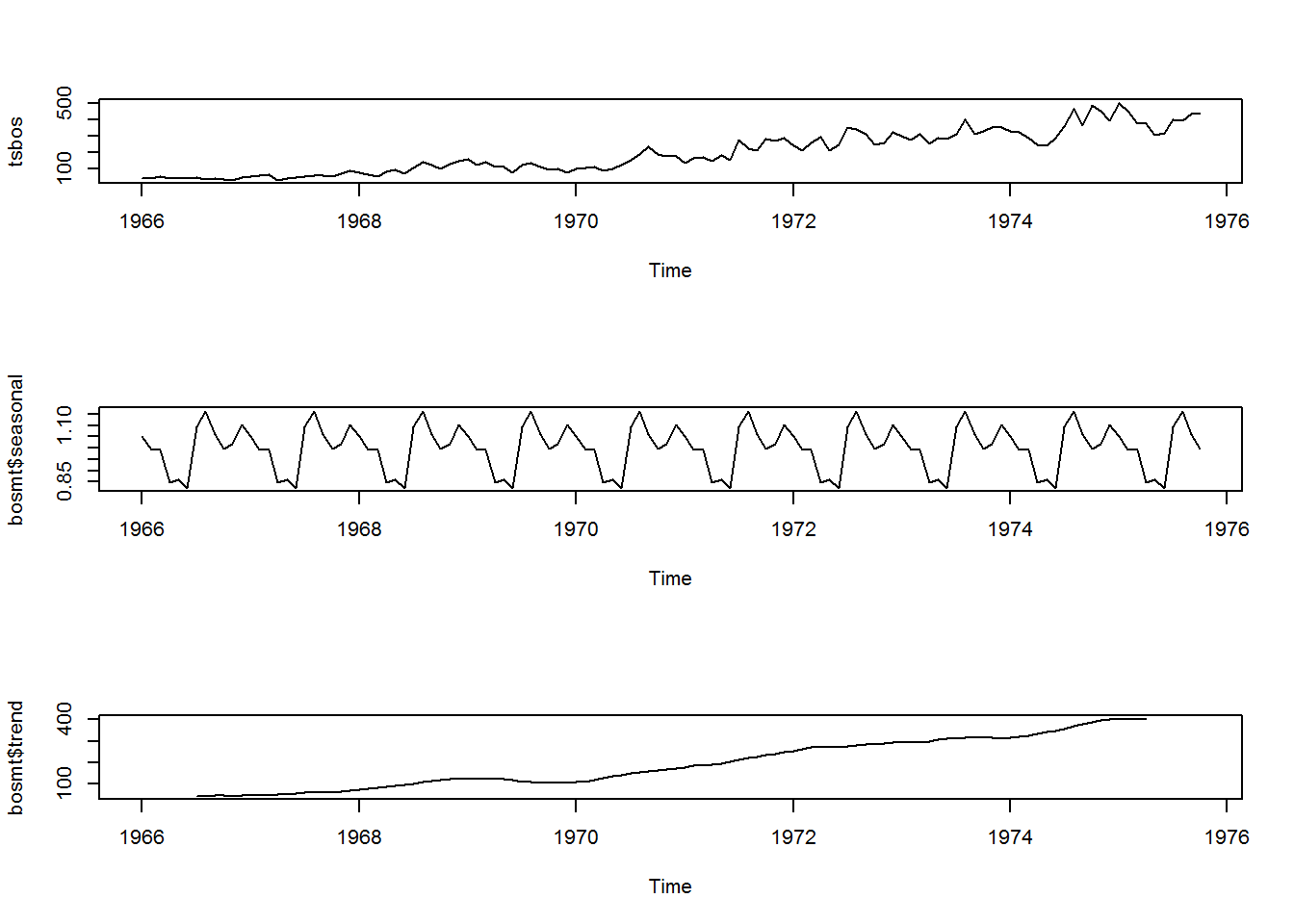``````### The above shows the trend and seasonal components of the multiplicative model

## We are multiplying these components in this model
predmt=bosmt\$trend * bosmt\$seasonal
residmt=tsbos - predmt
residbosmt = window(residmt,start = c(1966, 7), end = c(1975, 4))
rmsemt=sqrt(sum(residbosmt^2)/length(residbosmt))
rmsemt``````
``##  27.10955``
``````#### RMSE for multiplicative model is found to be 27.10, which is lower than the Additive Decomp model

layout(1:1)
plot(tsbos,lwd=2)
lines(predmt,col='red',lwd=2)``````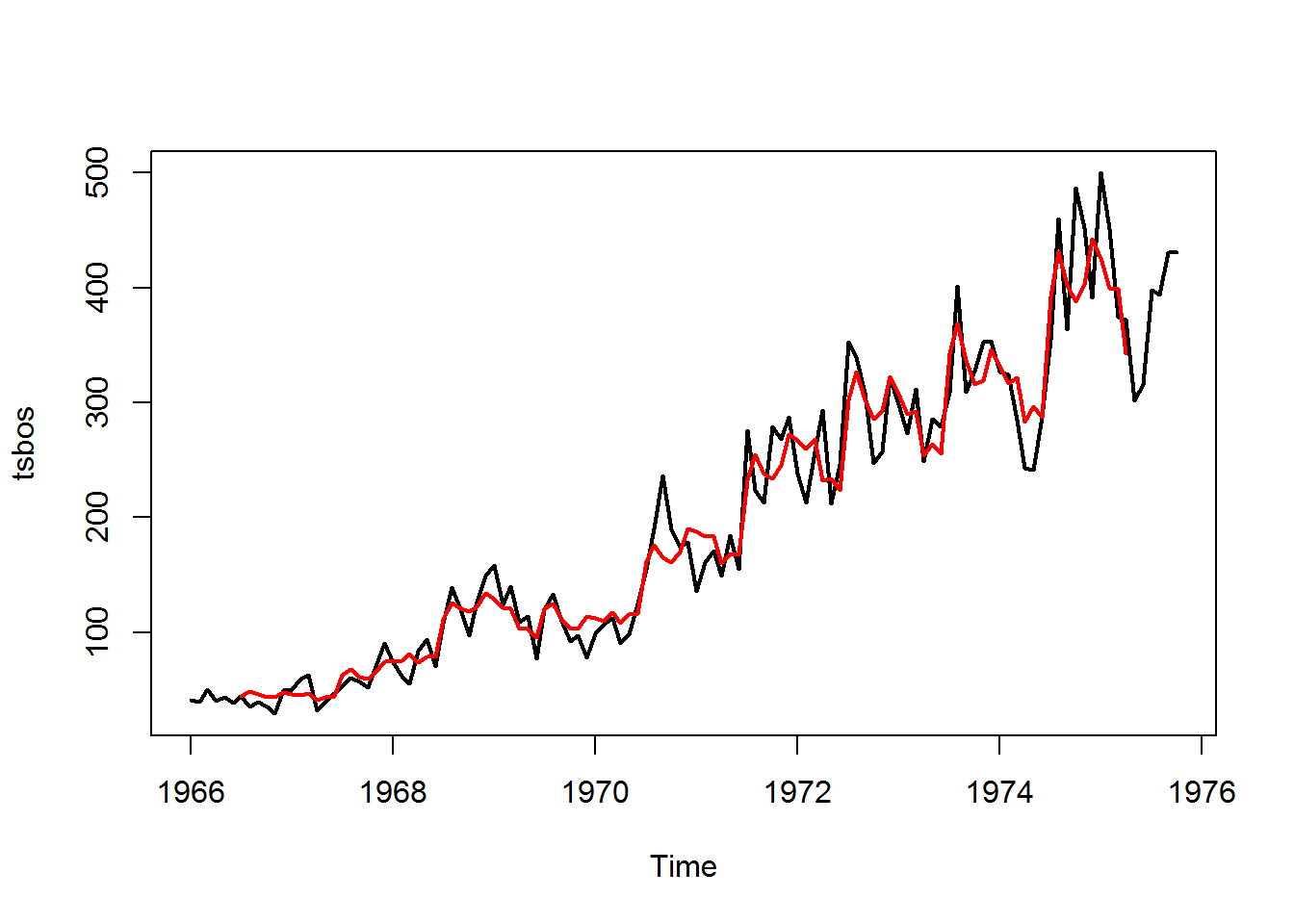``````#### The fitted lines looks to be doing well at the lower tail and better than the Additive Model at the higher tail

plot(bosmt\$random)``````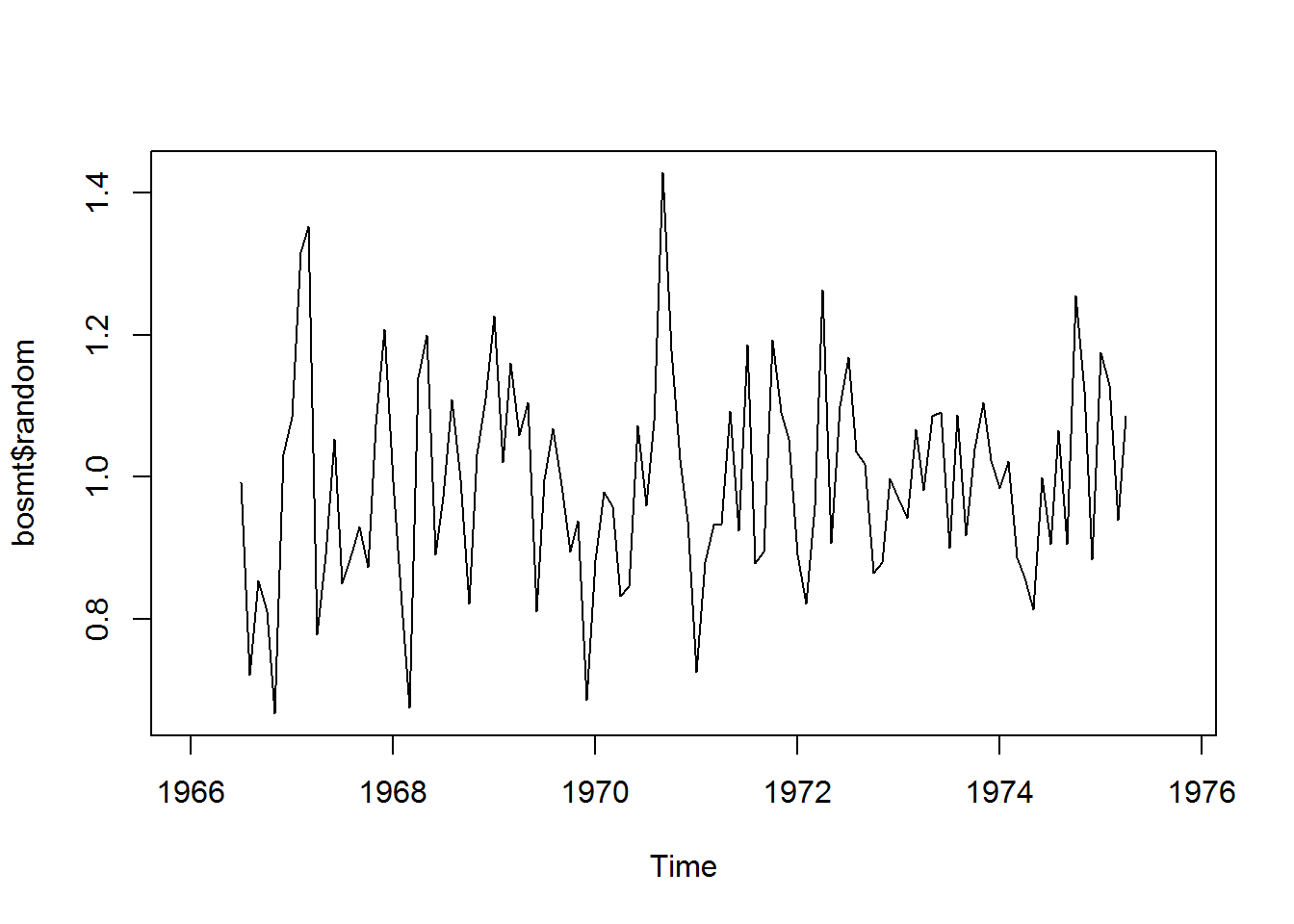``````plot(residbosmt)
abline(0,0)``````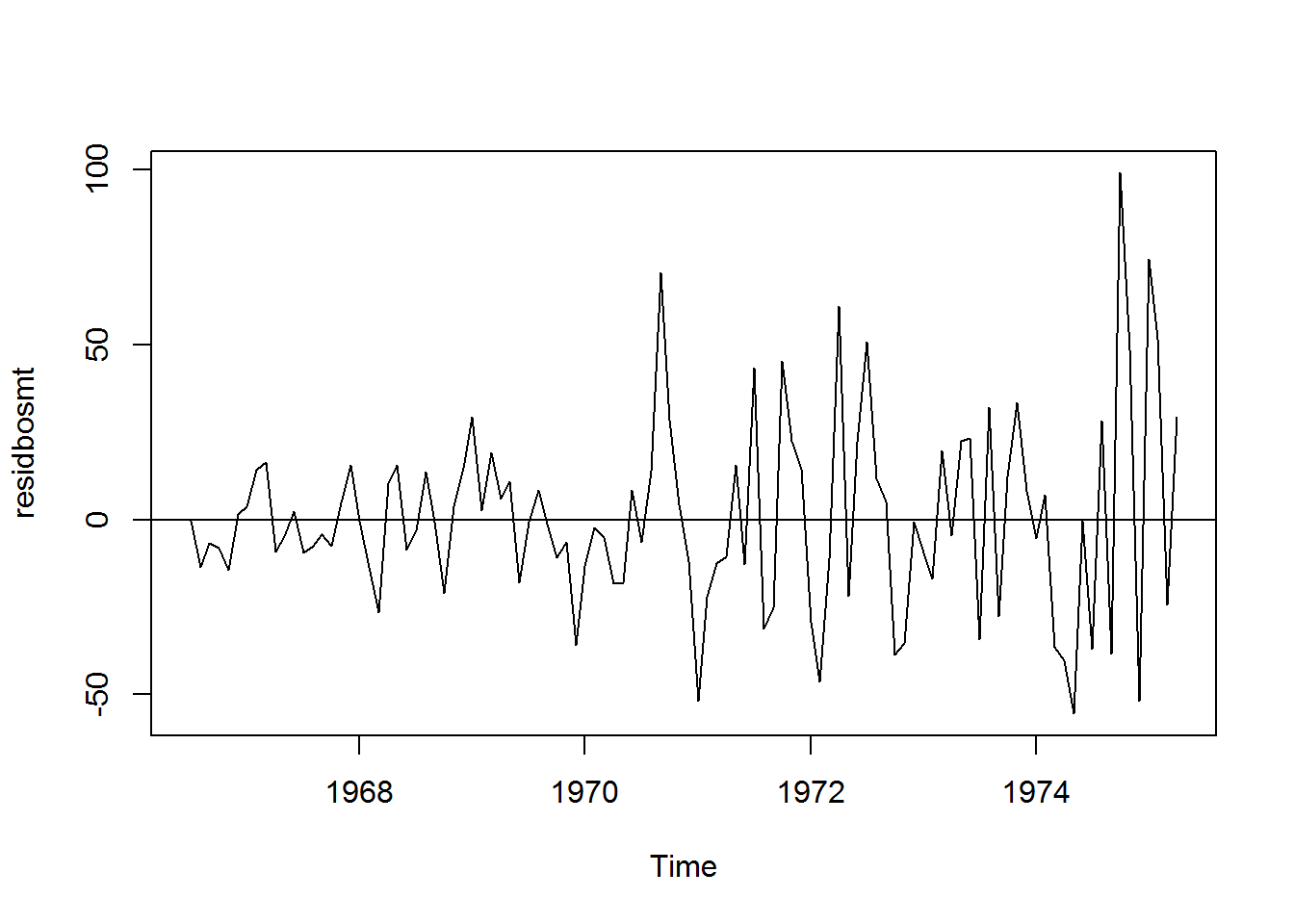``````#### Finalizing the Decomposition Moving Average Models ####
### After looking at the RMSE value (though there's no right value) and comparison of fitted lines & resuidal plots, its safe to say Multiplicative Decomposition has a better fit
## Hence let's use the mutiplicative model for forecasting

##### Fit the trend line
mttr=bosmt\$trend
plot(mttr)``````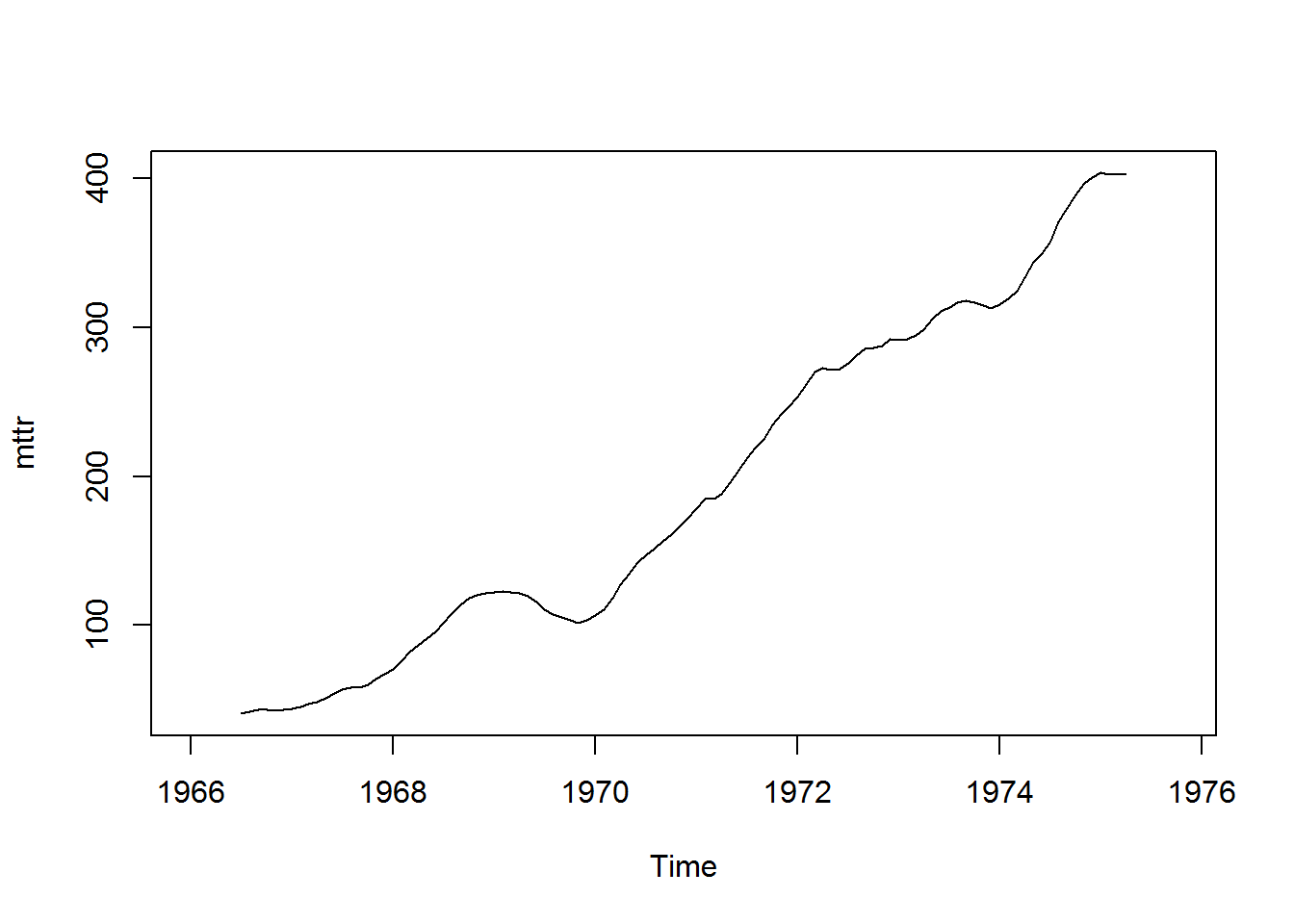``str(mttr)``
``##  Time-Series [1:118] from 1966 to 1976: NA NA NA NA NA ...``
``class(mttr)``
``##  "ts"``
``````timetr=time(mttr)

timr = unclass(timetr)

class(timr)``````
``##  "numeric"``
``````mtreg= lm(mttr~timr)

timwin=window(timr,start = c(1966, 7), end = c(1975, 4))

plot(mttr,lwd=2)
lines(timwin,fitted(mtreg),col='red',lwd=2)``````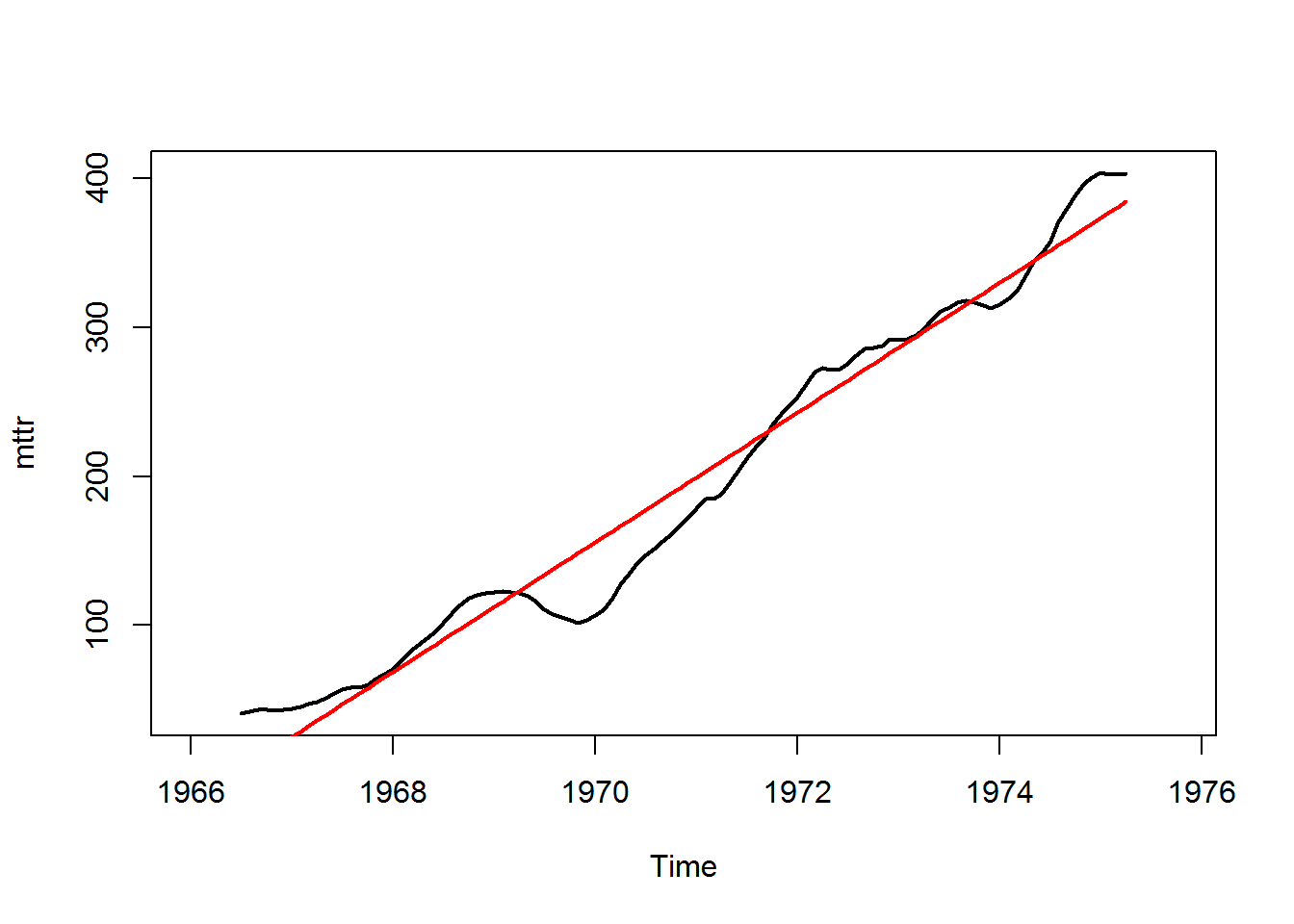``````timr=as.ts(timr)
summary(mtreg)``````
``````##
## Call:
## lm(formula = mttr ~ timr)
##
## Residuals:
##     Min      1Q  Median      3Q     Max
## -49.249 -13.466   5.194  13.493  37.282
##
## Coefficients:
##               Estimate Std. Error t value Pr(>|t|)
## (Intercept) -8.570e+04  1.560e+03  -54.95   <2e-16 ***
## timr         4.358e+01  7.913e-01   55.08   <2e-16 ***
## ---
## Signif. codes:  0 '***' 0.001 '**' 0.01 '*' 0.05 '.' 0.1 ' ' 1
##
## Residual standard error: 20.77 on 104 degrees of freedom
##   (12 observations deleted due to missingness)
## Multiple R-squared:  0.9669, Adjusted R-squared:  0.9665
## F-statistic:  3033 on 1 and 104 DF,  p-value: < 2.2e-16``````
``````### We are getting an adjusted R-Square value of 96.65 which is more that good.
### The pv-value of the F-test in regression suggests that all the variables in the model are significant
##### Prediction of 1975 Nov & Dec ####
forecastmt=predict(mtreg,data.frame(timr=c(1975.833,1975.917)),se.fit=TRUE)
forecastmt\$fit``````
``````##        1        2
## 409.9966 413.6573``````
``````### Adding the seasonal Component ###
semt=tail(bosmt\$seasonal)

forecastfinal=forecastmt\$fit+semt[5:6]

forecaster=forecastmt\$se.fit
forecastup=forecastfinal + 2 * forecaster
forecastlw=forecastfinal - 2 * forecaster
forecastup``````
``````##        1        2
## 419.8782 423.5956``````
``forecastlw``
``````##        1        2
## 402.2318 405.7123``````
``````#### Forecasts for November & December with 95% confidence interval are 420 to 402 & 424 to 406 respectively ####
######### END Of Question 1 ###############

#### Start of Question 2 ###################
##### LOESS decomposition model ####
# LOESS Model also uses both additive & Multiplicative methods for the fit
bosloess=stl(tsbos,s.window = "periodic")
str(bosloess)``````
``````## List of 8
##  \$ time.series: mts [1:118, 1:3] 6.8 -6.58 -8.46 -27.01 -34.46 ...
##   ..- attr(*, "dimnames")=List of 2
##   .. ..\$ : NULL
##   .. ..\$ : chr [1:3] "seasonal" "trend" "remainder"
##   ..- attr(*, "tsp")= num [1:3] 1966 1976 12
##   ..- attr(*, "class")= chr [1:3] "mts" "ts" "matrix"
##  \$ weights    : num [1:118] 1 1 1 1 1 1 1 1 1 1 ...
##  \$ call       : language stl(x = tsbos, s.window = "periodic")
##  \$ win        : Named num [1:3] 1181 19 13
##   ..- attr(*, "names")= chr [1:3] "s" "t" "l"
##  \$ deg        : Named int [1:3] 0 1 1
##   ..- attr(*, "names")= chr [1:3] "s" "t" "l"
##  \$ jump       : Named num [1:3] 119 2 2
##   ..- attr(*, "names")= chr [1:3] "s" "t" "l"
##  \$ inner      : int 2
##  \$ outer      : int 0
##  - attr(*, "class")= chr "stl"``````
``plot(bosloess)``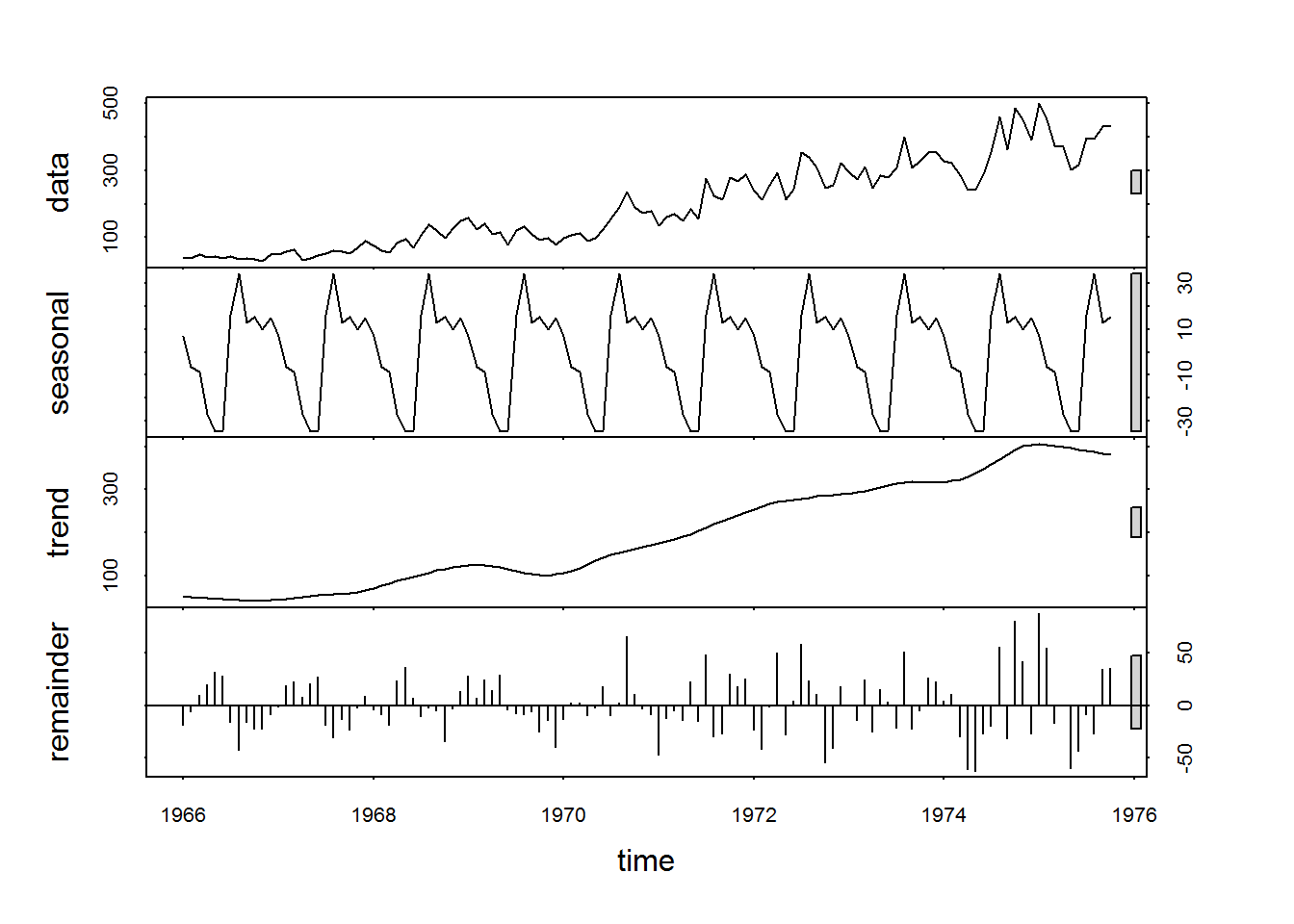``````### Plot indicates the presence of trend, seasonal & random elements in the data ####

#### We are starting our analysis witht the Stl additive model ####
pred1=bosloess\$time.series[,'seasonal'] + bosloess\$time.series[,'trend']
plot(tsbos,lwd=2)
lines(pred1,col='red',lwd=2)``````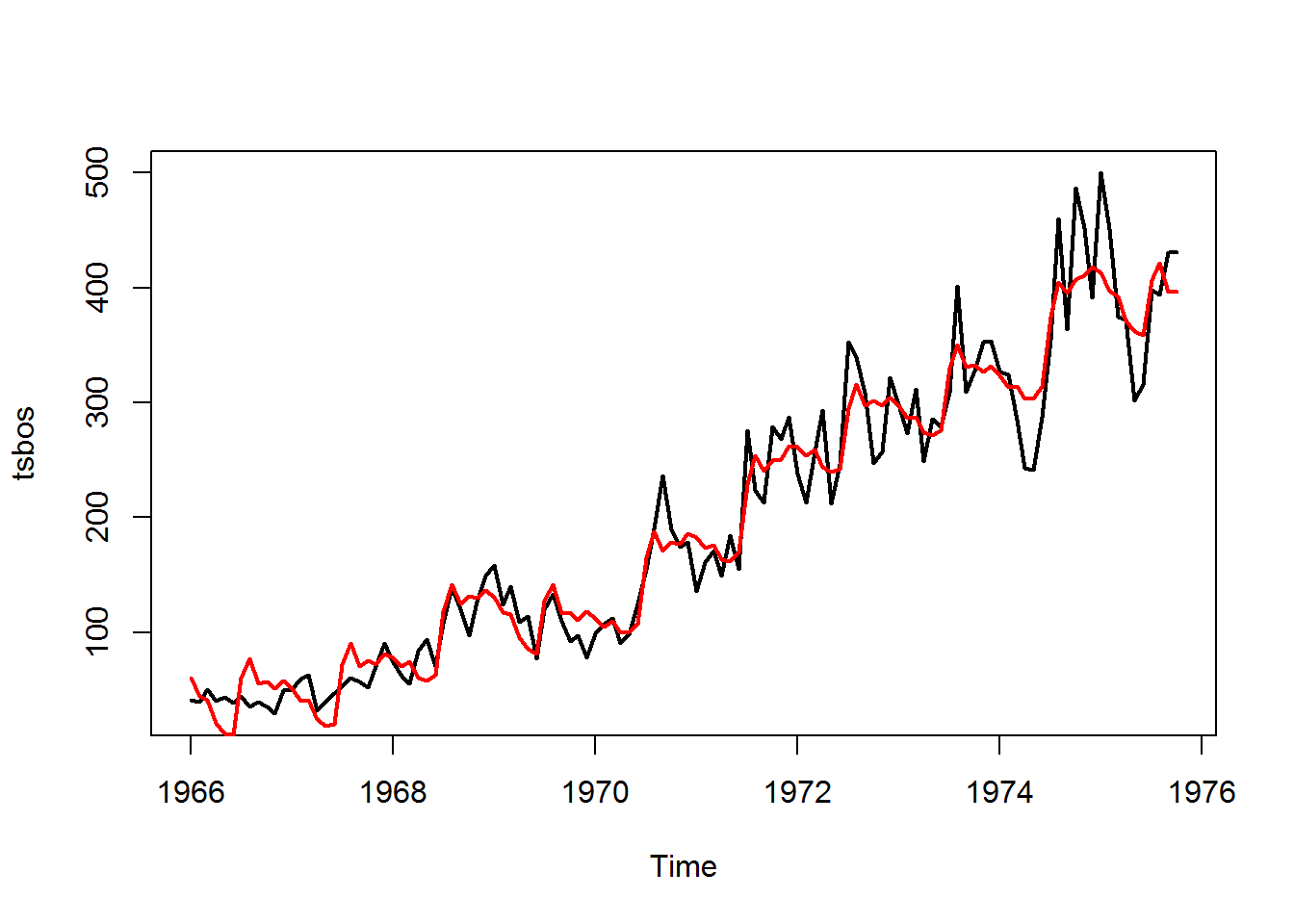``````##### fitted line seems to be doing badly at both tails
##### missing the peaks as the trend increases (Overestimating at the lower tail & Underestimating at the higher tail)

rmse1=sqrt(sum(bosloess\$time.series[,'remainder']^2)/length(bosloess\$time.series[,'remainder']))
rmse1``````
``##  28.47619``
``````#### rmse for loess additive is 28.47 #####

#### visualizing the remainder values ####
plot(bosloess\$time.series[,'remainder'],ylab='REMAINDER')
abline(0,0)``````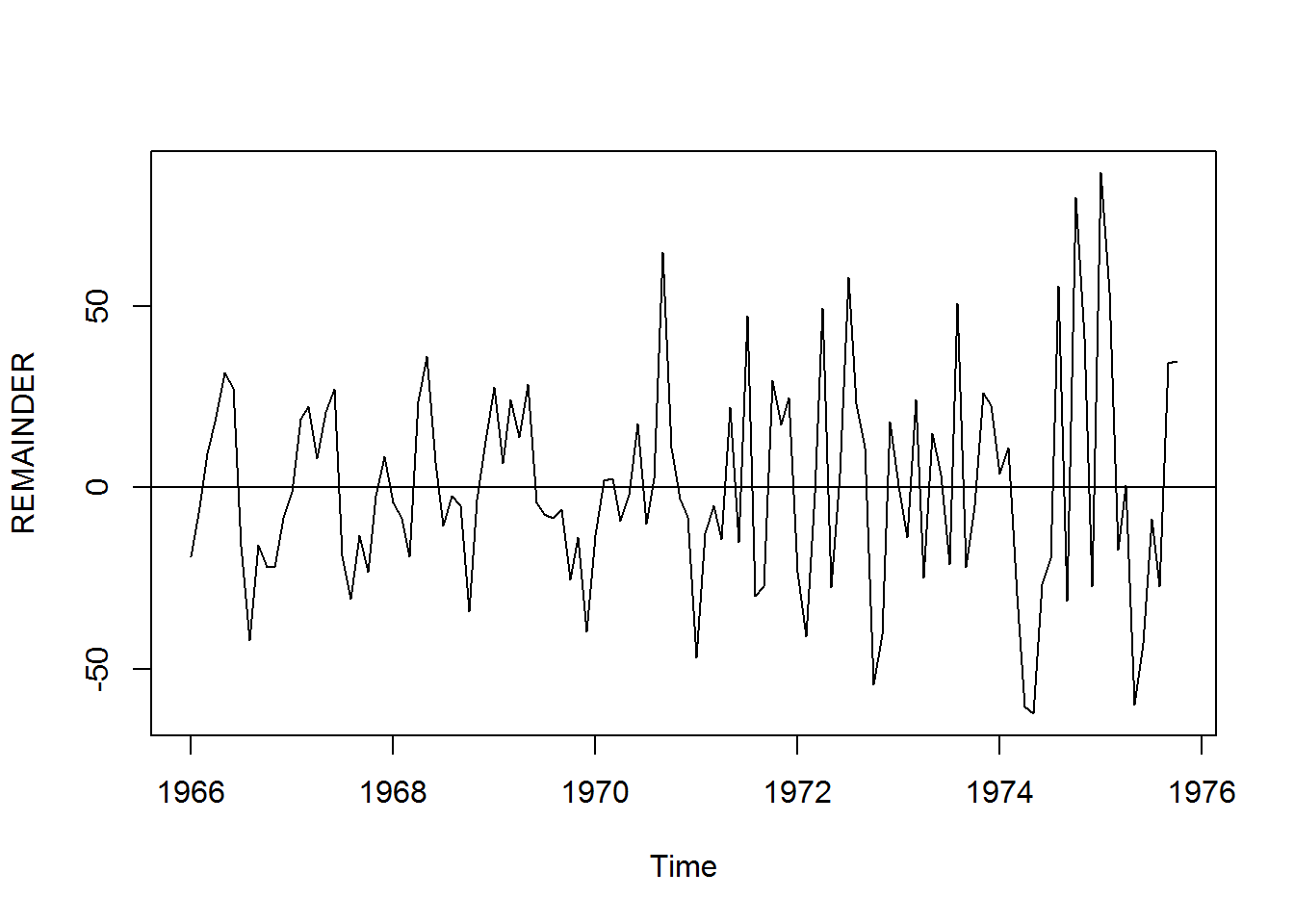``````#### Indicates the variance seem to be slightly increasing as the time increases

#### LOESS multiplicative model ####
## When using the LOESS multiplicative model we apply the natural log to turn the method into a additive model

logbos=log(tsbos)

plot(logbos,ylab='log(boston robbery)')``````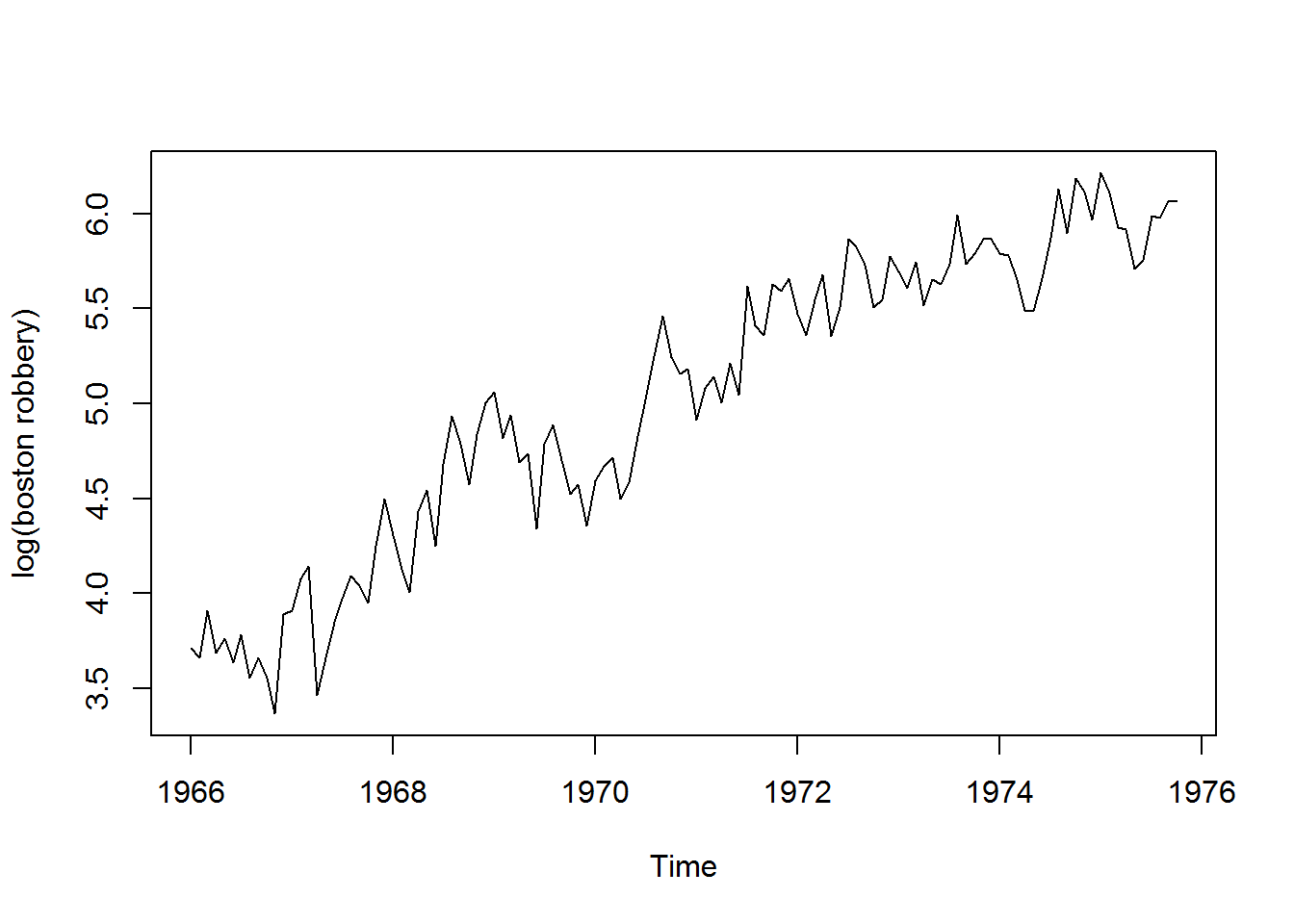``````### Plot shows the distribution of the log(robbery data)
class(logbos)``````
``##  "ts"``
``````boslog=stl(logbos,s.window = 'periodic')
plot(boslog)``````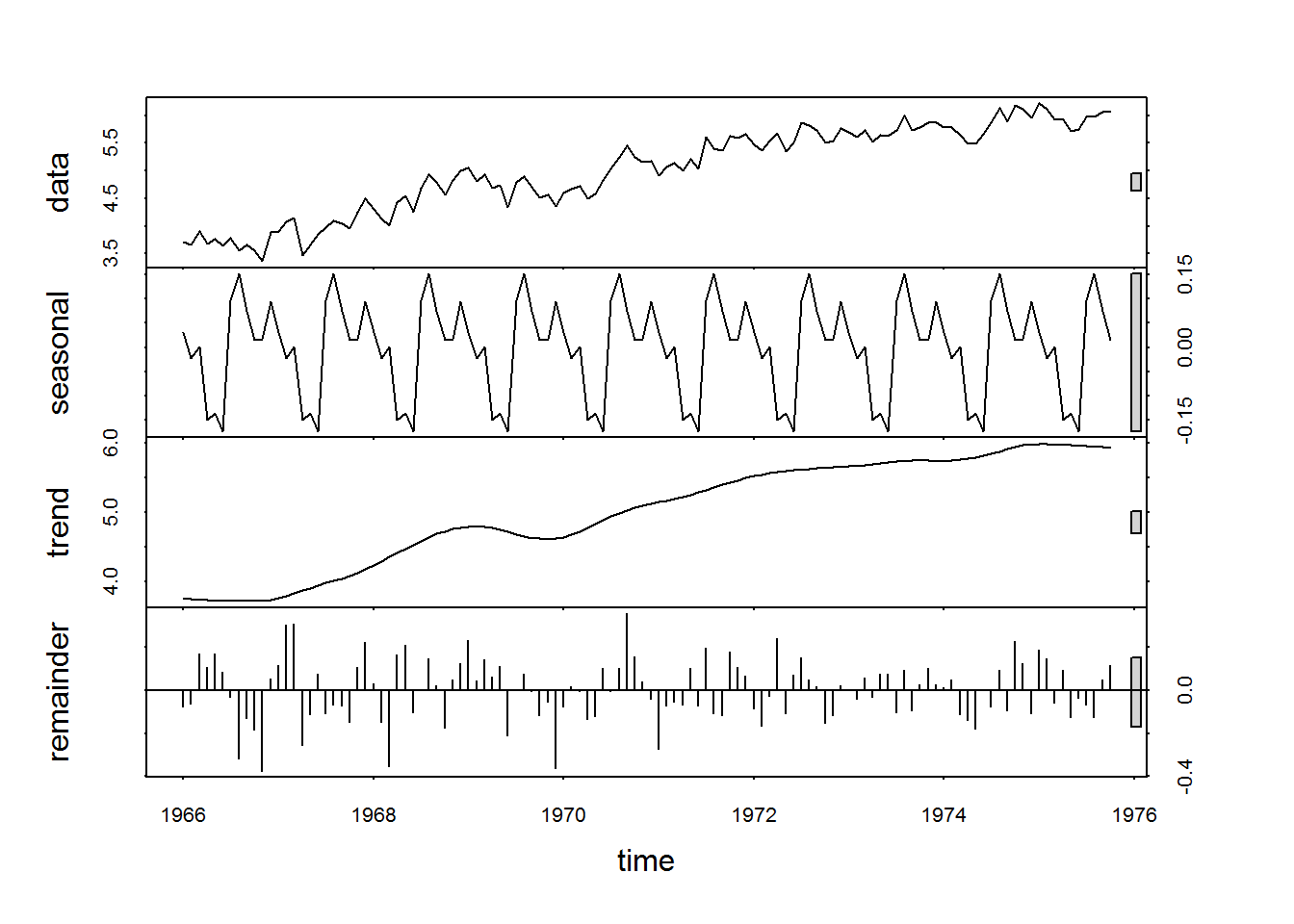``````## Plot indicates the seasonal, trend & random elements in the data
#### seasonality seems to be constant over the time period and trend is linearly positive

pred2=boslog\$time.series[,'seasonal'] + boslog\$time.series[,'trend']
## We are adding seasonal & trend component because of the natural log
plot(logbos,lwd=1)
lines(pred2,col='red',lwd=3)``````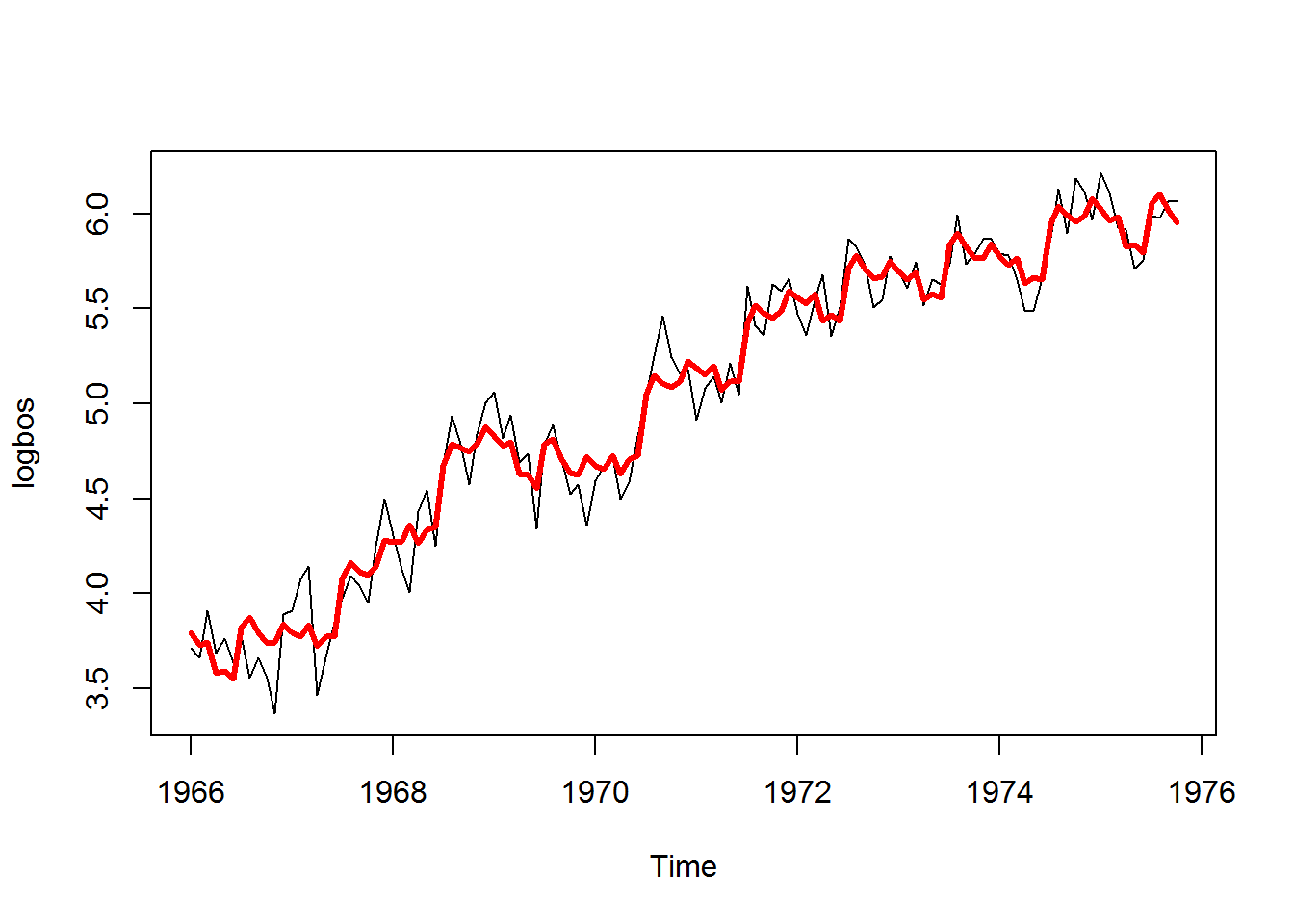``````#### Fitted lines doesn't seem to fit well on the seasonal higher & lower peaks, We can see slight underestimation in the fit

## calculating the RMSE ####
residlg=tsbos - exp(pred2)
rsmelog=sqrt(sum(residlg^2)/length(residlg))
rsmelog``````
``##  27.0506``
``````#### RSME for LOESS Multiplicative model is 27.0506 #####
#### Looking at the both the models(LOESS addictive & LOESS Multiplicative), the multiplicative one has the better RSME and fitted plot looks better as well, hence it's logical to choose the multiplicative model for forecast

## Forecasting for the last two months ##
trlogmt=boslog\$time.series[,'trend']
plot(trlogmt)``````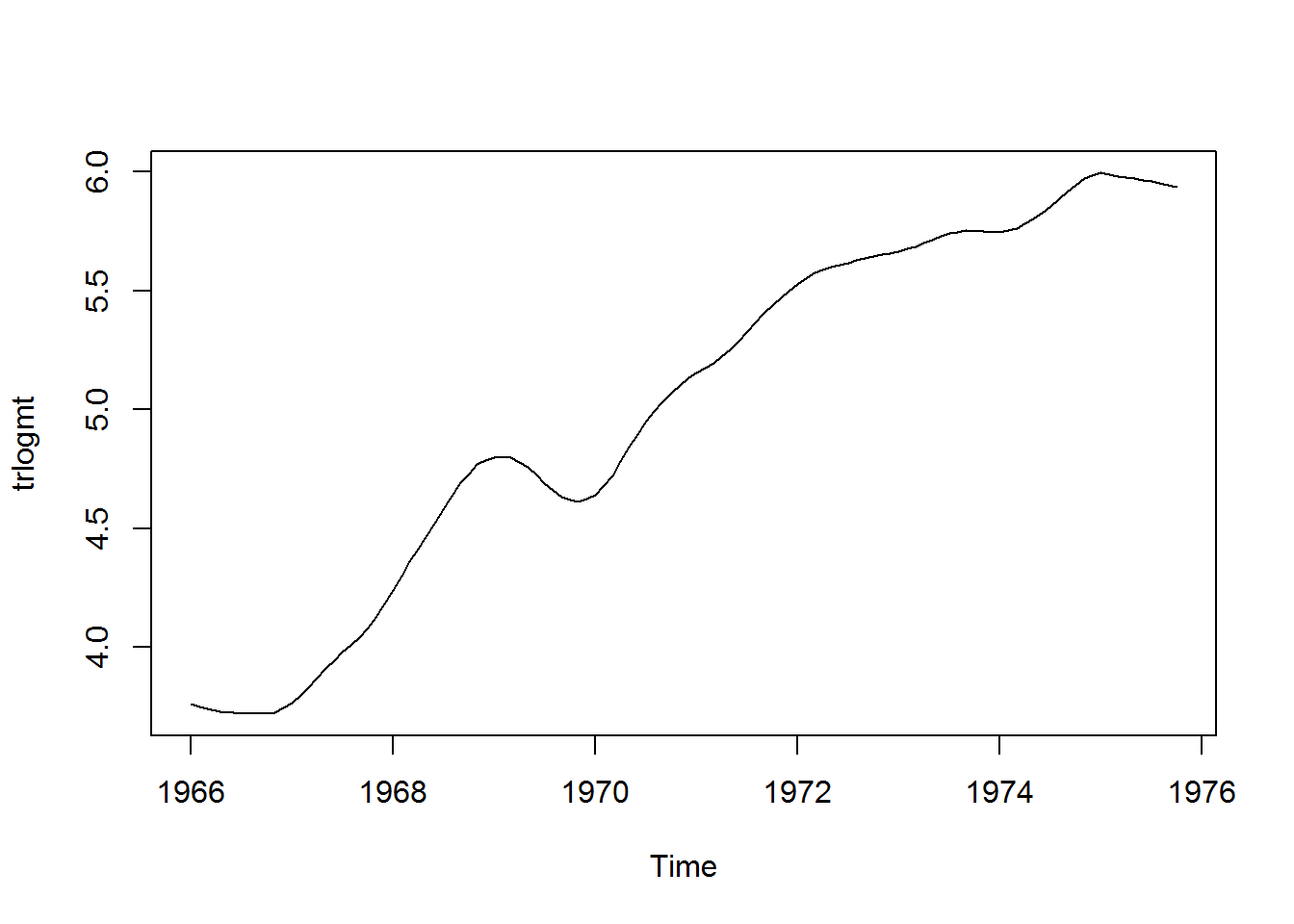``````#### There seems to be a positive trend with the increase in time, but we can see a dip in robberies just before the year 1970

str(trlogmt)``````
``##  Time-Series [1:118] from 1966 to 1976: 3.76 3.75 3.74 3.73 3.73 ...``
``class(trlogmt)``
``##  "ts"``
``````timelogmt=time(trlogmt)

timelgmt=unclass(timelogmt)

logmtreg=lm(trlogmt~timelgmt)
summary(logmtreg)``````
``````##
## Call:
## lm(formula = trlogmt ~ timelgmt)
##
## Residuals:
##      Min       1Q   Median       3Q      Max
## -0.34574 -0.11822 -0.00523  0.12403  0.28454
##
## Coefficients:
##               Estimate Std. Error t value Pr(>|t|)
## (Intercept) -5.067e+02  1.003e+01  -50.52   <2e-16 ***
## timelgmt     2.597e-01  5.089e-03   51.02   <2e-16 ***
## ---
## Signif. codes:  0 '***' 0.001 '**' 0.01 '*' 0.05 '.' 0.1 ' ' 1
##
## Residual standard error: 0.1569 on 116 degrees of freedom
## Multiple R-squared:  0.9573, Adjusted R-squared:  0.957
## F-statistic:  2603 on 1 and 116 DF,  p-value: < 2.2e-16``````
``````#### We get a good Adjusted R-squared of 95.7% & p-value is very small hence the variables are significant

plot(trlogmt,lwd=2)
lines(timelgmt,fitted(logmtreg),col='red',lwd=2)``````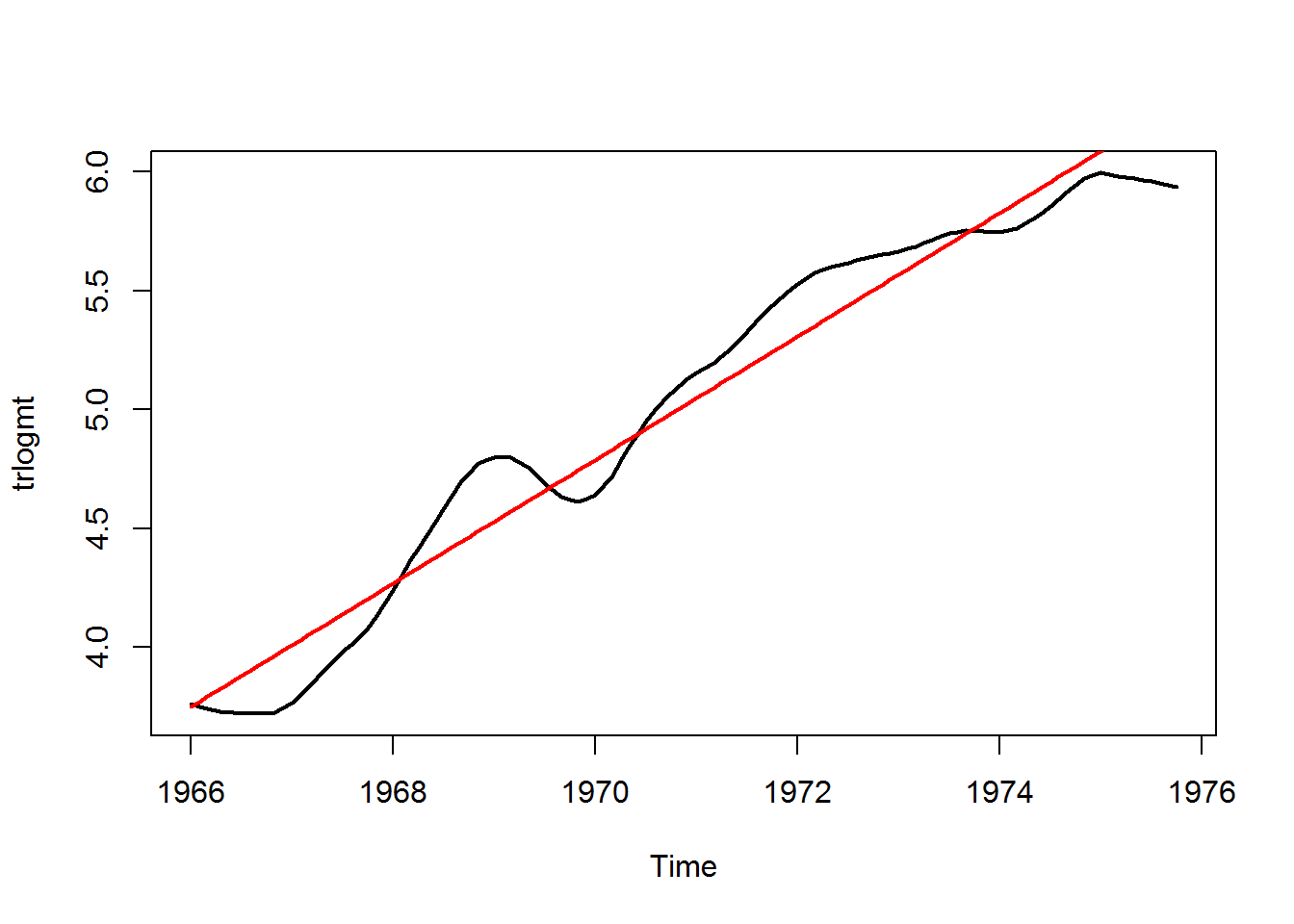``````#### Forecasting for the logmultiplicative model ####
predmt=predict(logmtreg,data.frame(timelgmt=c(1975.833,1975.917)),se.fit = TRUE)
predmt``````
``````## \$fit
##        1        2
## 6.303092 6.324903
##
## \$se.fit
##          1          2
## 0.02907656 0.02944834
##
## \$df
##  116
##
## \$residual.scale
##  0.1569314``````
``````##### Adding the seasonality value into the predicted values ####
seas=tail(boslog\$time.series[,'seasonal'])
predval=predmt\$fit + seas[5:6]
predval``````
``````##        1        2
## 6.377526 6.340839``````
``````prederlg=predmt\$se.fit
prederlgup=predval + 2*prederlg
prederlglw=predval - 2*prederlg

forecastlogmtup=exp(prederlgup)
forecastlogmtlw=exp(prederlglw)
forecastlogmtup``````
``````##        1        2
## 623.7061 601.6857``````
``forecastlogmtlw``
``````##        1        2
## 555.2248 534.8262``````
``````##### 95% confidence intervals for the predicted armed robberies in Nov 1975 are 624 and 555, whereas for Dec 1975, they are 602 and 535.  ####

##### End of Question 2 #####

##### Start of Question 3  (Linear Regression Model) #######

### Here we would be using function of time to represent the trend component and the seasona using dummy variables as factors ###

ltime=time(tsbos)
ltime``````
``````##           Jan      Feb      Mar      Apr      May      Jun      Jul
## 1966 1966.000 1966.083 1966.167 1966.250 1966.333 1966.417 1966.500
## 1967 1967.000 1967.083 1967.167 1967.250 1967.333 1967.417 1967.500
## 1968 1968.000 1968.083 1968.167 1968.250 1968.333 1968.417 1968.500
## 1969 1969.000 1969.083 1969.167 1969.250 1969.333 1969.417 1969.500
## 1970 1970.000 1970.083 1970.167 1970.250 1970.333 1970.417 1970.500
## 1971 1971.000 1971.083 1971.167 1971.250 1971.333 1971.417 1971.500
## 1972 1972.000 1972.083 1972.167 1972.250 1972.333 1972.417 1972.500
## 1973 1973.000 1973.083 1973.167 1973.250 1973.333 1973.417 1973.500
## 1974 1974.000 1974.083 1974.167 1974.250 1974.333 1974.417 1974.500
## 1975 1975.000 1975.083 1975.167 1975.250 1975.333 1975.417 1975.500
##           Aug      Sep      Oct      Nov      Dec
## 1966 1966.583 1966.667 1966.750 1966.833 1966.917
## 1967 1967.583 1967.667 1967.750 1967.833 1967.917
## 1968 1968.583 1968.667 1968.750 1968.833 1968.917
## 1969 1969.583 1969.667 1969.750 1969.833 1969.917
## 1970 1970.583 1970.667 1970.750 1970.833 1970.917
## 1971 1971.583 1971.667 1971.750 1971.833 1971.917
## 1972 1972.583 1972.667 1972.750 1972.833 1972.917
## 1973 1973.583 1973.667 1973.750 1973.833 1973.917
## 1974 1974.583 1974.667 1974.750 1974.833 1974.917
## 1975 1975.583 1975.667 1975.750``````
``````lseas=cycle(tsbos)
ldata=coredata(tsbos)
ldata``````
``````##     41  39  50  40  43  38  44  35  39  35  29  49  50  59  63  32  39
##    47  53  60  57  52  70  90  74  62  55  84  94  70 108 139 120  97
##   126 149 158 124 140 109 114  77 120 133 110  92  97  78  99 107 112
##    90  98 125 155 190 236 189 174 178 136 161 171 149 184 155 276 224
##   213 279 268 287 238 213 257 293 212 246 353 339 308 247 257 322 298
##   273 312 249 286 279 309 401 309 328 353 354 327 324 285 243 241 287
##  355 460 364 487 452 391 500 451 375 372 302 316 398 394 431 431``````
``lseas``
``````##      Jan Feb Mar Apr May Jun Jul Aug Sep Oct Nov Dec
## 1966   1   2   3   4   5   6   7   8   9  10  11  12
## 1967   1   2   3   4   5   6   7   8   9  10  11  12
## 1968   1   2   3   4   5   6   7   8   9  10  11  12
## 1969   1   2   3   4   5   6   7   8   9  10  11  12
## 1970   1   2   3   4   5   6   7   8   9  10  11  12
## 1971   1   2   3   4   5   6   7   8   9  10  11  12
## 1972   1   2   3   4   5   6   7   8   9  10  11  12
## 1973   1   2   3   4   5   6   7   8   9  10  11  12
## 1974   1   2   3   4   5   6   7   8   9  10  11  12
## 1975   1   2   3   4   5   6   7   8   9  10``````
``````### Extracting the seasonality, time & the core data from the time series dataset

## Regression function ##
lnreg=lm(ldata~0+ltime+factor(lseas))
summary(lnreg)``````
``````##
## Call:
## lm(formula = ldata ~ 0 + ltime + factor(lseas))
##
## Residuals:
##     Min      1Q  Median      3Q     Max
## -90.911 -28.339  -0.222  23.333 119.000
##
## Coefficients:
##                   Estimate Std. Error t value Pr(>|t|)
## ltime               41.978      1.349   31.11   <2e-16 ***
## factor(lseas)1  -82525.111   2658.540  -31.04   <2e-16 ***
## factor(lseas)2  -82539.409   2658.652  -31.05   <2e-16 ***
## factor(lseas)3  -82542.207   2658.765  -31.05   <2e-16 ***
## factor(lseas)4  -82561.606   2658.877  -31.05   <2e-16 ***
## factor(lseas)5  -82569.904   2658.989  -31.05   <2e-16 ***
## factor(lseas)6  -82570.702   2659.102  -31.05   <2e-16 ***
## factor(lseas)7  -82521.100   2659.214  -31.03   <2e-16 ***
## factor(lseas)8  -82504.198   2659.327  -31.02   <2e-16 ***
## factor(lseas)9  -82526.496   2659.439  -31.03   <2e-16 ***
## factor(lseas)10 -82524.994   2659.552  -31.03   <2e-16 ***
## factor(lseas)11 -82528.315   2658.993  -31.04   <2e-16 ***
## factor(lseas)12 -82523.813   2659.106  -31.03   <2e-16 ***
## ---
## Signif. codes:  0 '***' 0.001 '**' 0.01 '*' 0.05 '.' 0.1 ' ' 1
##
## Residual standard error: 41.47 on 105 degrees of freedom
## Multiple R-squared:  0.9721, Adjusted R-squared:  0.9686
## F-statistic:   281 on 13 and 105 DF,  p-value: < 2.2e-16``````
``````### Adjusted R Square is 96.86 which is a good value and the P-value indicates that time variable is sufficient to predict the robberies in Boston
plot(lnreg)``````